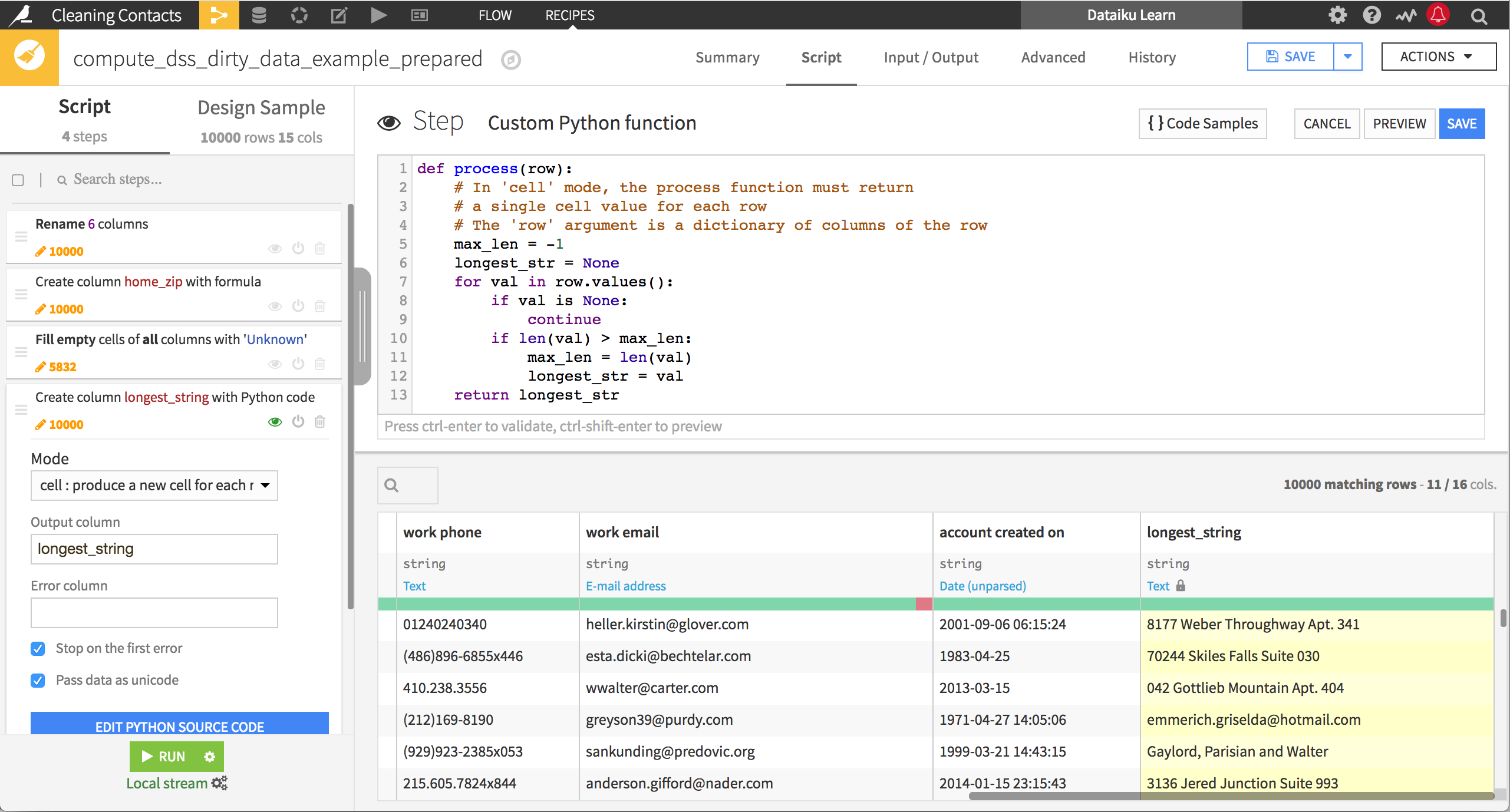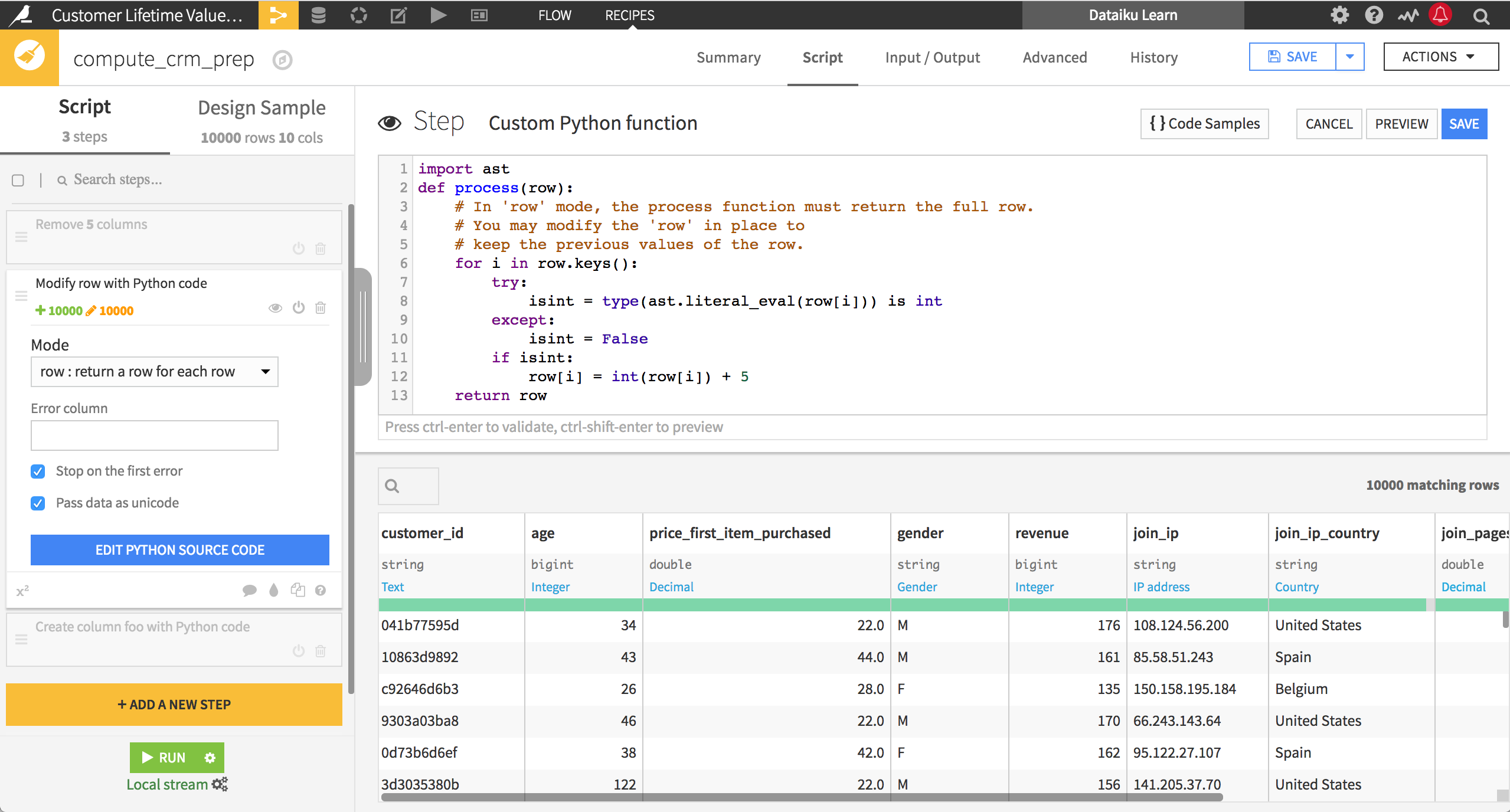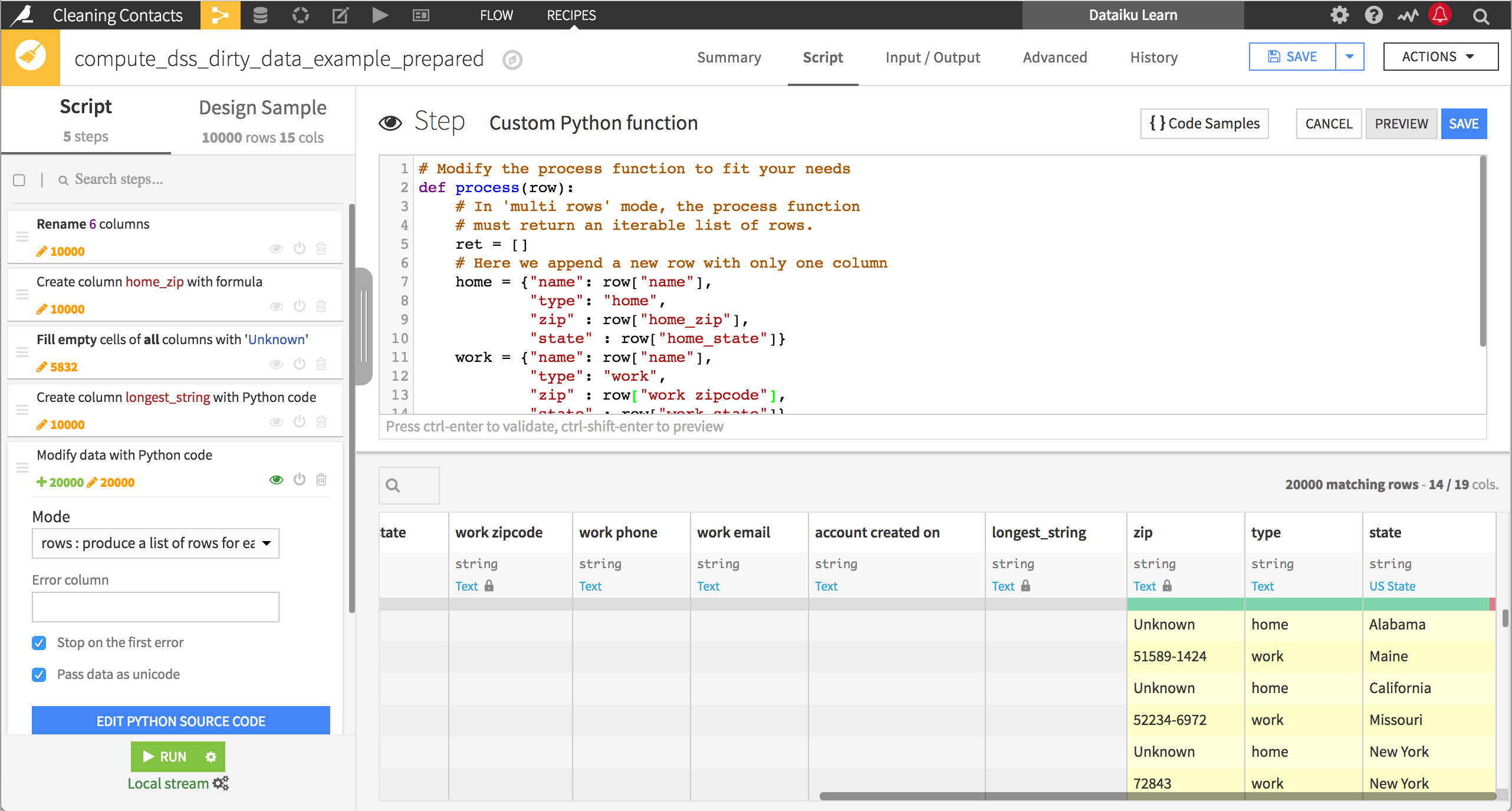# Reference | Using custom Python functions in the Prepare recipe¶

The Prepare recipe holds processors to handle the most common data preparation tasks. For more customized tasks, you can craft operations in the Formula language.

For even more complex tasks, or according to your preference, you can also use the Python function processor.

This processor allows you to perform complex row-wise operations on your dataset. The output of the function depends on which of the three modes is used:

Mode

Returns

Cell

A new cell for each row

Row

A row for each row

Rows

A list of rows for each row## Cell mode¶

In cell mode, Dataiku expects a single column as output. This is useful for finding the largest value across columns, or the sum of a set of columns whose names follow some pattern.

In the example below, the process() function finds the longest string value across the columns in the dataset.

def process(row):
# In 'cell' mode, the process function must return
# a single cell value for each row
# The 'row' argument is a dictionary of columns of the row
max_len = -1
longest_str = None
for val in row.values():
if val is None:
continue
if len(val) > max_len:
max_len = len(val)
longest_str = val
return longest_str## Row mode¶

In row mode, Dataiku expects a row as output, which replaces the existing row. This is useful when you need to perform operations in place on the rows (as in the image below), or when you need to add multiple rows to the dataset (such as if you compute the mean and standard deviation of values in a row across columns).

In the example below, the process() function adds 5 to the integer-valued columns. Note that the row parameter passed to the process() function is a dictionary of the string values, so we use the ast package to evaluate whether they are integer-valued.

import ast
def process(row):
# In 'row' mode, the process function must return the full row.
# You may modify the 'row' in place to
# keep the previous values of the row.
for i in row.keys():
try:
isint = type(ast.literal_eval(row[i])) is int
except:
isint = False
if isint:
row[i] = int(row[i]) + 5
return row## Rows mode¶

In rows mode, Dataiku expects one or more rows as output, which replace the existing row. This is especially useful when you need to transform your data from wide to long format.

In the example below, the process() function splits each row into two. Where before a single row contained both a person’s work and home zip code and state, now each row contains either the home or work information, along with a new column that indicates whether it is the home or work information.

# Modify the process function to fit your needs
def process(row):
# In 'multi rows' mode, the process function
# must return an iterable list of rows.

ret = []

home = {"name": row["name"],
"type": "home",
"zip": row["home_zip"],
"state": row["home_state"]}

work = {"name": row["name"],
"type": "work",
"zip": row["work zipcode"],
"state": row["work state"]}

ret.append(home)
ret.append(work)

return ret